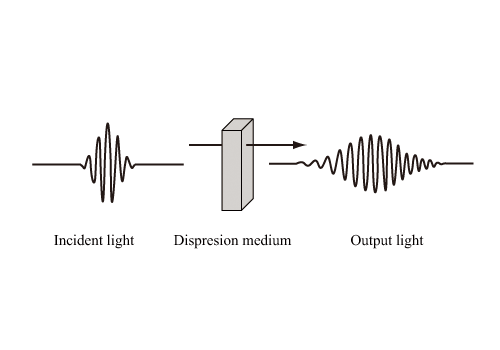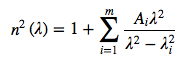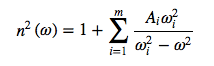The wavelength dispersion is a wavelength-dependent delay of light propagation time. When an optical pulse is affected by the wavelength dispersion, the pulse duration is elongated. Fig.2.14 schematically explains the dispersion effect on optical pulse penetrating through a dispersive medium.Figure 2.14: Schematic of dispersion

Generally, “dispersion” means the wavelength dispersion, but not a mode dispersion and a polarized wave mode dispersion, although these are also optical fiber dispersions.

The wavelength dispersion is divided into a material dispersion due to a refractive index change in a material and a waveguide dispersion due to a waveguide structure. The wavelength dispersion is the the sum of material dispersion and waveguide dispersion. The material dispersion can not be controlled since it is inherent to a material itself (quartz glass, etc.). On the other hand, the waveguide dispersion is controlled by changing the core radius, the relative refractive index difference, and the the refractive index distribution.

The wavelength dispersion is yielded by a frequency (wavelength) dependency of refractive index of an inductive medium. A propagation velocity of light in a medium with a refractive index of n(λ) is expressed as c/n(λ) where n is the light velocity in air. This means that the light velocity propagating in the medium is varied dependent on a wavelength of light.

According to the Sellmeier equation, derived by W.Sellmeier in 1871, a refractive index of medium, n(λ), is expressed by the following equation,where Ai is an intensity of light at a resonant frequency, νi, λi is given as λi=c/νi. Ai, λi, and m are constants depending on a material, and are estimated in experiments.

The Sellmeier equation can not give an accurate result in the vicinity of ν0 since it diverges at ν0. Excluding the frequency range, however, it approximates the wavelength dispersion with a good accuracy (for m=3, in the range of 365-2325 nm, the precision of refractive index calculation is ±5×10−6). The constants (Ai， λi) for various media can be obtained from “Handbook of Optical Constants of Solids” by E.D.Palik. For silica glass, at room temperature (18℃) and m=3, A1=0.6961663, A2=0.4079426, A3=0.8974794, λ1=0.0684043, λ2=0.1162414, λ3=9.896161 are known, and the refractive index becomes lower as the wavelength becomes longer.

Eq.17 is rewritten as a function of frequency, n(ω), which is expressed by the following equation,where ωi equals to 2πc/λi.